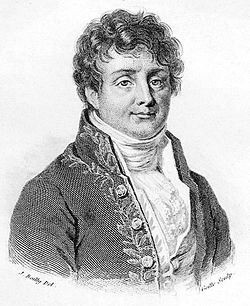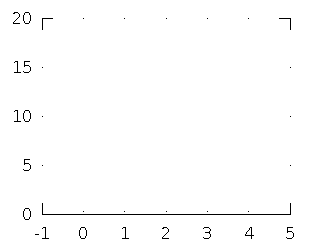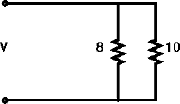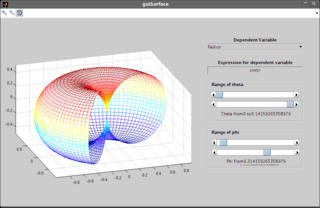# math

## Polynomial Interpolation (Lagrange Interpolation) with Vandermonde Matrix &#x2013; Matlab / GNU Octave Code

May 16, 2017

A quick but ill-conditioned way to compute a Lagrange polynomial interpolation…

Tags: math, matlab, octave

## Fourier Reconstruction of an Image

Dec 11, 2014Any image can be reconstructed using just a superposition of plane waves of different spatial frequencies. This is a numerical experiment using a NumPy script that reconstructs an image one frequency component at a time.

Tags: math, signals, software

## Convolution and Polynomial Multiplication

Mar 26, 2012

"Convolution in the time domain is multiplication in the frequency domain" is an oft-quoted statement. But the exact relation between the two is seldom stressed adequately. Mathematically, convolution and polynomial multiplication are one and the same process, as I shall explain shortly.

Tags: math, signals

## How does convolution work?

Mar 26, 2012The process of convolution works on the twin concepts of linearity and shift invariance. Here is an animated explanation of the concept.

Tags: math, signals

## Modular Exponentiation

Mar 26, 2012

Modular exponentiation is essentially a technique used to calculate c=b

Tags: math

## Polynomials are Numbers!

Mar 26, 2012

Polynomials are numbers of an unknown base x…

Tags: math

## Quantitative Aptitude for the Electrical Engineer

Mar 26, 2012This is based on my dissatisfaction with the messed up way time and work problems are handled in quantitative aptitude examination guides. They take a simple idea, threaten you with time constraints and leave you feeling broken and guilty because you couldn't solve them quickly enough.

Tags: math

## Throughput of Aloha Networks

Mar 26, 2012

A derivation of the mathematical expression for the throughput of an aloha network using the exponential and Poisson random distributions

Tags: math

## Lagrange Interpolation - Matlab / GNU Octave Code

Mar 18, 2012

This piece of code is a Matlab/GNU Octave function to perform Lagrange interpolation.

Tags: math, matlab, octave

## Spherical Coordinates Matlet

Mar 17, 2012This "matlet" is work we did for an assignment we had in the third semester on Electromagnetic Fields. The problem statement was to create an applet which could plot surfaces in the spherical coordinate system.

Tags: math, matlab, software